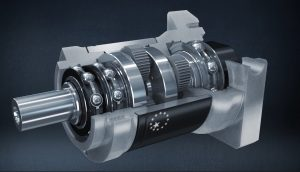Back to the overview

# Economy PII series gearboxes available with gear ratio 12:1Gearboxes from the Economy PII Series from Apex Dynamics are per immediately available with a 12:1 transmission ratio. It is the 16th gear ratio for the popular series and even 24th for the PGII variant. Nevertheless, this is a welcome addition for various applications. In this article we explain the PII series and explain when this gear ratio comes in useful.

## Gearboxes with 12:1 ratio

Apex Dynamics can now supply the entire Economy PII Series, straight and right-angled, with a gear ratio of 12:1. The PII series consists of:

• PEII: The most common size with round B14 flange
• PGII: As PEII with slightly different dimensions, with round B14 flange
• PAII: Dimensions as the AB series, with square B5 flange
• PSII: As PAII with slightly different dimensions, with square B5 flange
• PN: Dimensions as NEMA, with square B5 flange
• PD: Dimensioning according to ISO9409.
• PL: Output side suitable for a timing belt pulley.

## Why 12:1 gear ratio?

After the already extensive gear ratios of 3, 4, 5, 7, 10, 15, 16, 20, 25, 30, 35, 40, 50, 70 and 100 (plus 120, 160, 200, 280,350, 500 , 700 and 1000 for the PGII series) add another ratio? Why would you want another ratio between 10 and 15?

The gear ratio 12 is convenient in some applications because you can avoid rounding differences in continuously running applications. You can divide 360 degrees by 12 and you get a whole number: 360 / 12 = 30. And 30 degrees is a commonly used mathematical angle, as are 60 and 90 degrees. In addition, ratio 12 is one of the strongest gear ratios.

## Importance of the gear ratio in gear unitsThe term “gear ratio” in the context of gearboxes refers to the ratio between the input and output speeds of the system. In simple terms, if a gearbox has a gear ratio of 12:1, it means that for every 12 revolutions of the input (driving) gear, the output (driven) gear makes one complete revolution.

This is often used to reduce speed and increase torque. In this particular example of a 12:1 gear ratio, the input gear would turn relatively fast, while the output gear would turn much slower. But the torque – or turning force – on the output gear would be 12 times higher than on the input gear (assuming no energy loss from friction or other factors).

This principle is a fundamental basis of many machines and vehicles, from car engines to wind turbines and industrial machines. It allows us to control and adapt the energy generated by an engine or other power source to the specific demands of the task needed.

## Supply of PII series gearboxes

The delivery time for gearboxes with these new gear ratios is as fast as ever and the prices are the same as those of existing gear ratios. Tell us more about your application or challenge us and we will respond quickly with a substantive response. You will almost always receive a quote within one working day, mostly within in a few hours. Contact us at sales@apexdyna.nl for more information.

GearboxesNewsbevel gearclean2antarcticaFood Grade lubricantgearboxgearboxeshypoid gearboxeslinear drivelow backlashpinionrackservo motorstainless steelteamrembrandtsenUniversity Racing EindhovenUniversity Racing Eindhoven Formula Student teamWoTS